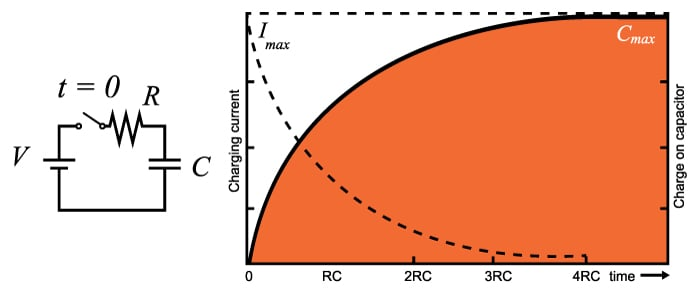# Capacitor Charge and Time Constant Calculator

## This calculator computes for the capacitor charge time and energy, given the supply voltage and the added series resistance.

V
μF
Ω

Joules
seconds

### Calculating Energy Stored in a Capacitor

This calculator is designed to compute for the value of the energy stored in a capacitor given its capacitance value and the voltage across it. The time constant can also be computed if a resistance value is given. Note that the input capacitance must be in microfarads (μF).### Equations

$$E = \frac{CV^{2}}{2}$$

$$\tau = RC$$

Where:

$$V$$ = applied voltage to the capacitor (volts)

$$C$$ = capacitance (farads)

$$R$$ = resistance (ohms)

$$\tau$$ = time constant (seconds)

The time constant of a resistor-capacitor series combination is defined as the time it takes for the capacitor to deplete 36.8% (for a discharging circuit) of its charge or the time it takes to reach 63.2% (for a charging circuit) of its maximum charge capacity given that it has no initial charge. The time constant also defines the response of the circuit to a step (or constant) voltage input. Consequently, the cutoff frequency of the circuit is defined by the time constant.

### Charging/Discharging Applications

The charging/discharging property of a capacitor made a lot of applications in electrical engineering possible. Here are some of them:

#### Flash Lamp

The flash lamp of a throwaway camera is powered by the charge stored on a capacitor. The circuit of a flash lamp normally consists of a large high-voltage polarized electrolytic capacitor to store the necessary charge, a flash lamp to generate the required light, a 1.5-v battery, a chopper network to generate a dc voltage in excess of 300 V, and a trigger network to establish a few thousand volts for a very short period of time to fire the flash lamp. It should certainly be of some interest that a single source of energy of only 1.5 V dc can be converted to one of a few thousand volts (albeit for a very short period of time) to fire the flash lamp. In fact, that single, small battery has sufficient power for the entire run of film through the camera.

#### Surge Protector

In recent years we have all become familiar with the line conditioner as a safety measure for our computers, TVs, CD players, and other sensitive instrumentation. In addition to protecting equipment from unexpected surges in voltage and current, most quality units will also filter out (remove) electromagnetic interference (EMI) and radio-frequency interference (RFI). The filtering is done with the right combination of a resistor and a capacitor. The charging and discharging of the capacitor means it would not allow rapid voltage spikes that would otherwise harm appliances and equipment.